DLARON 旷野森林香水包装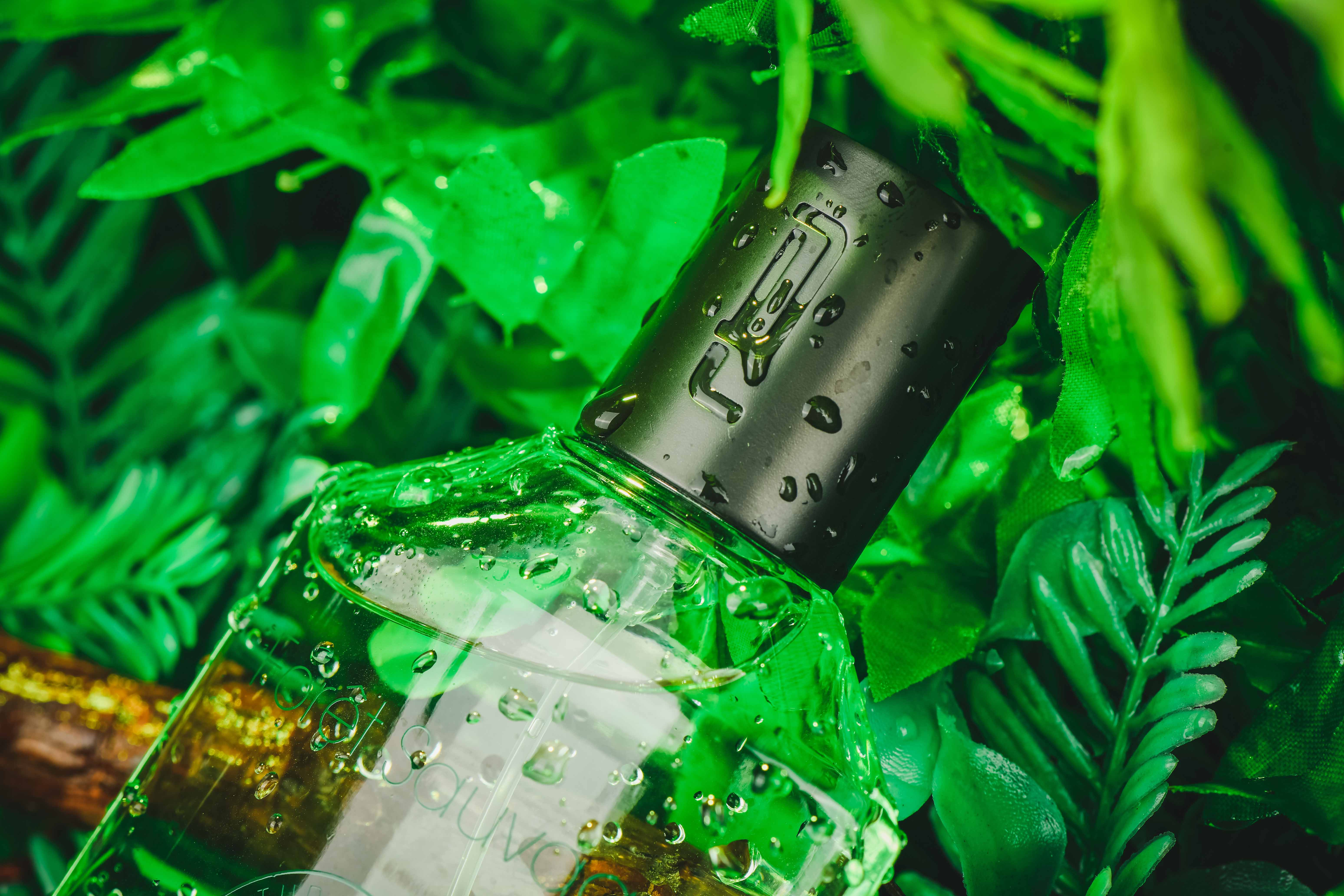HARUKA机油品牌设计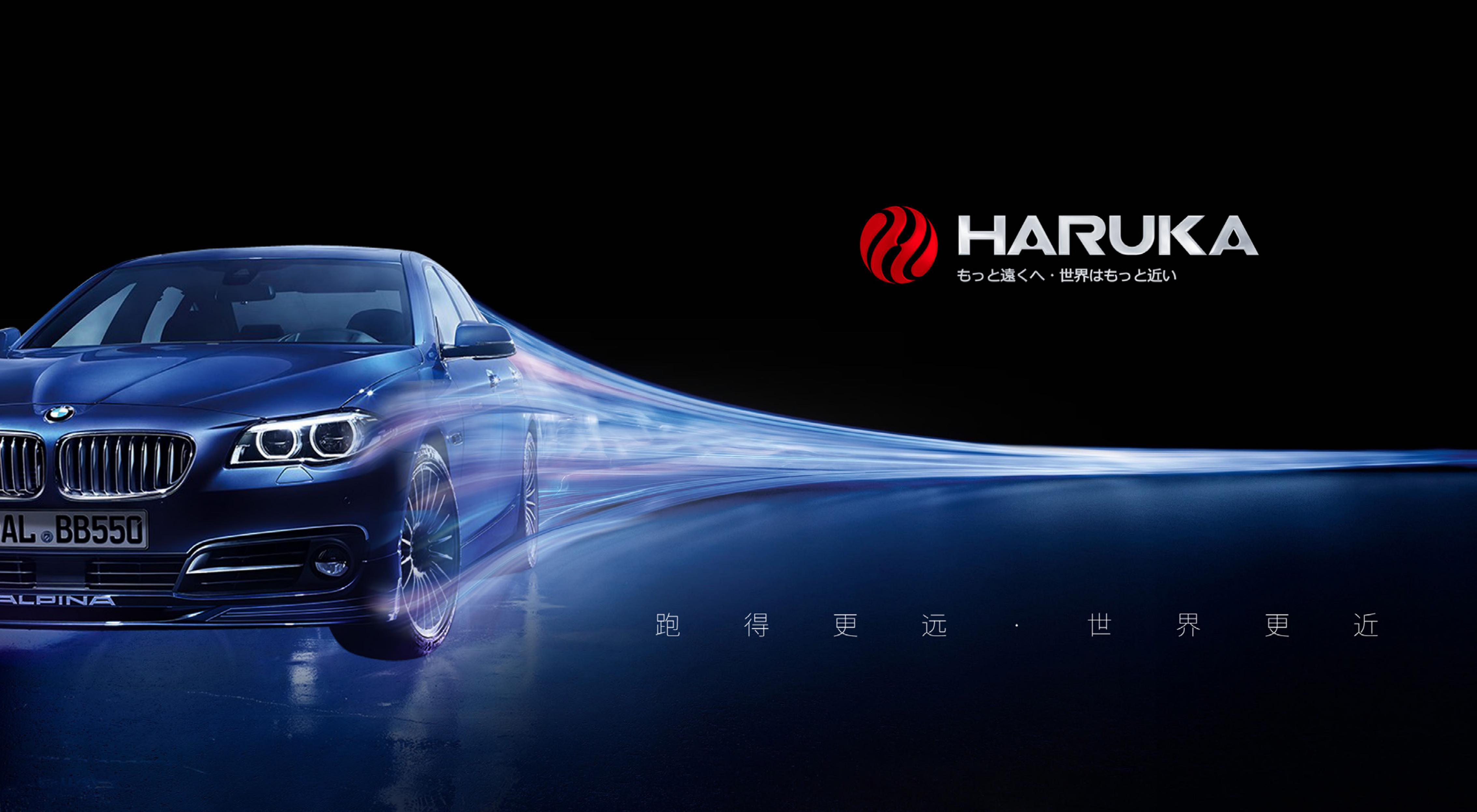DLARON 海蓝之谜香水包装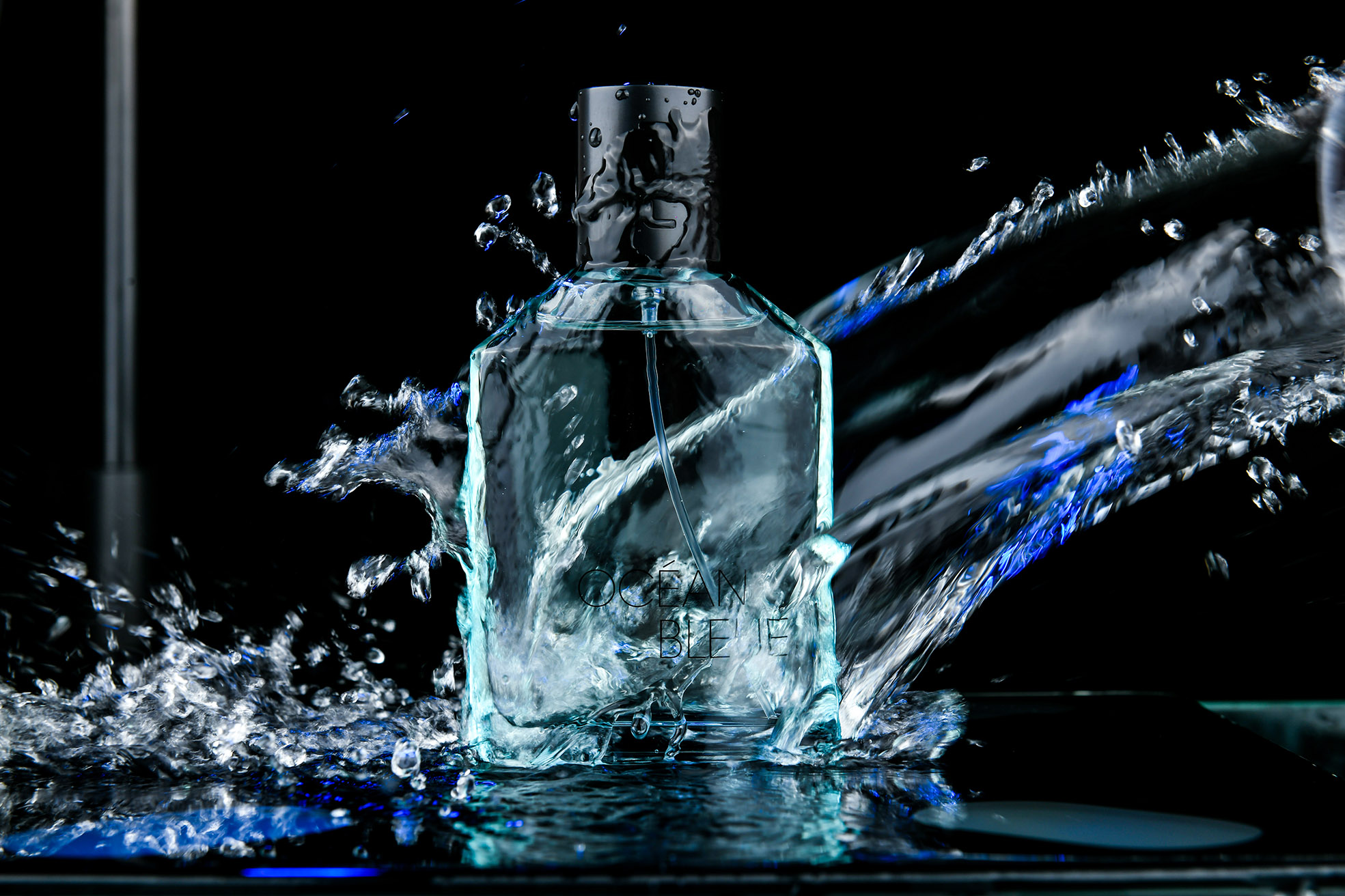DLARON幻想曲详香水包装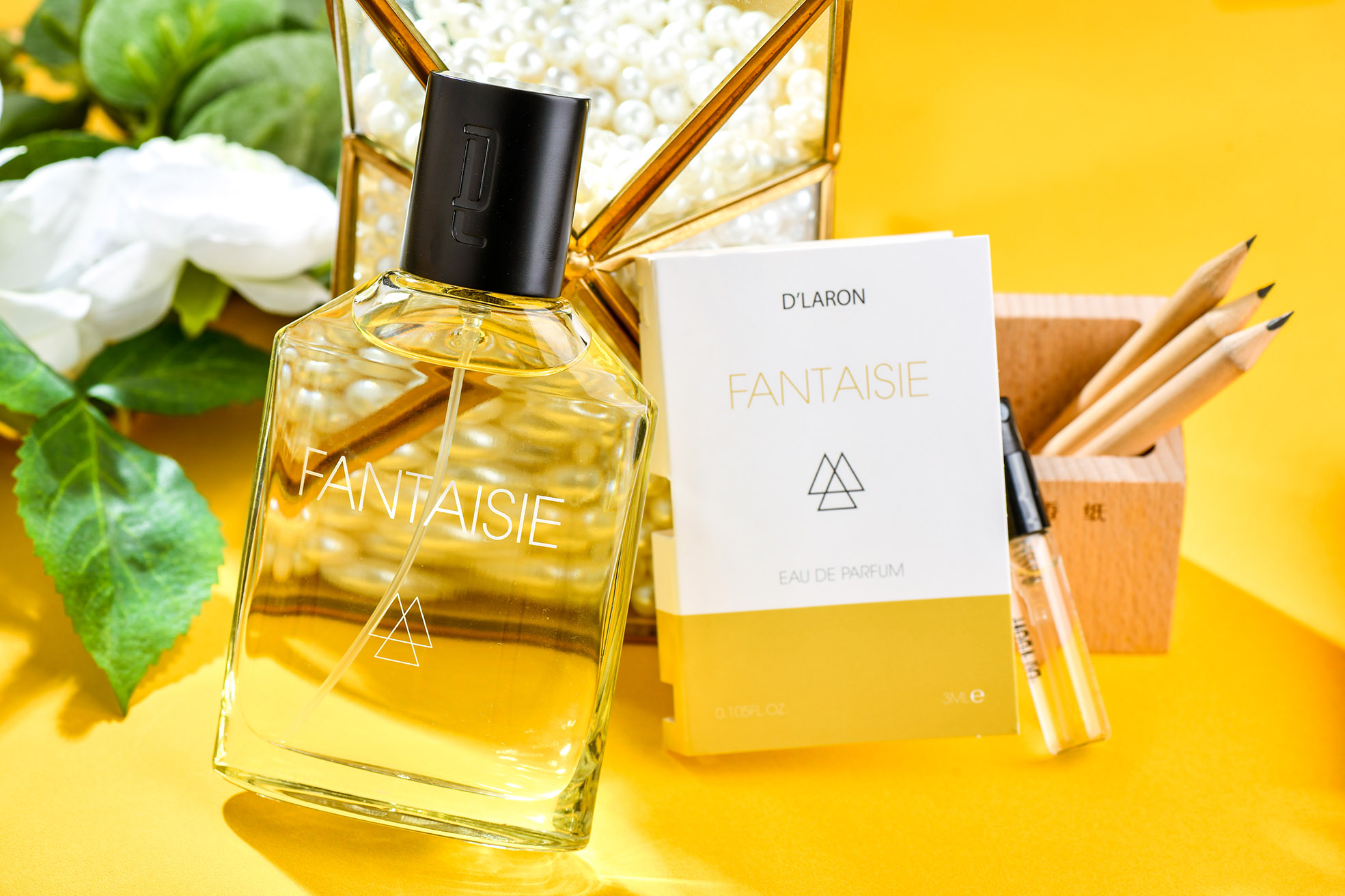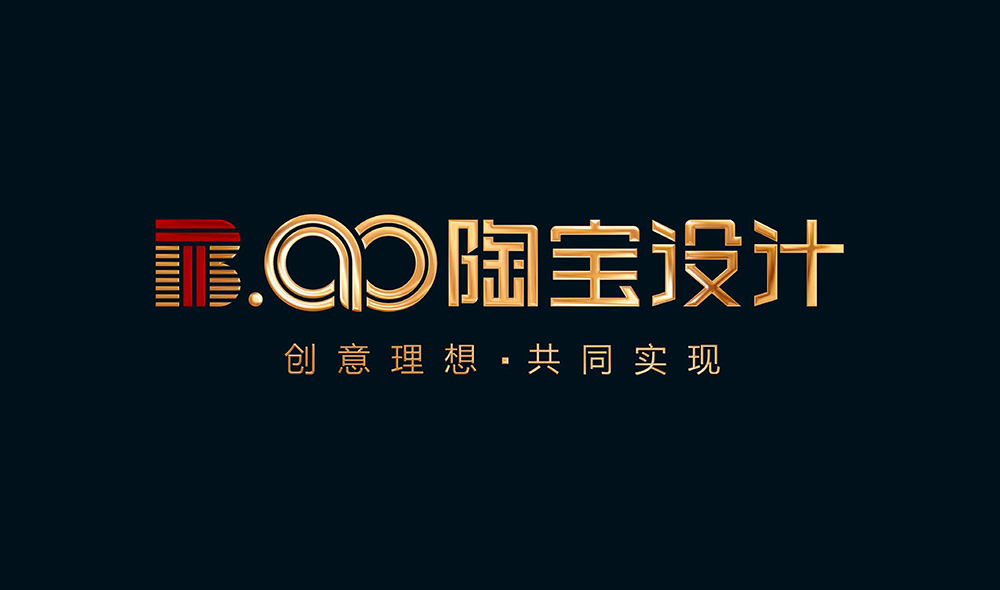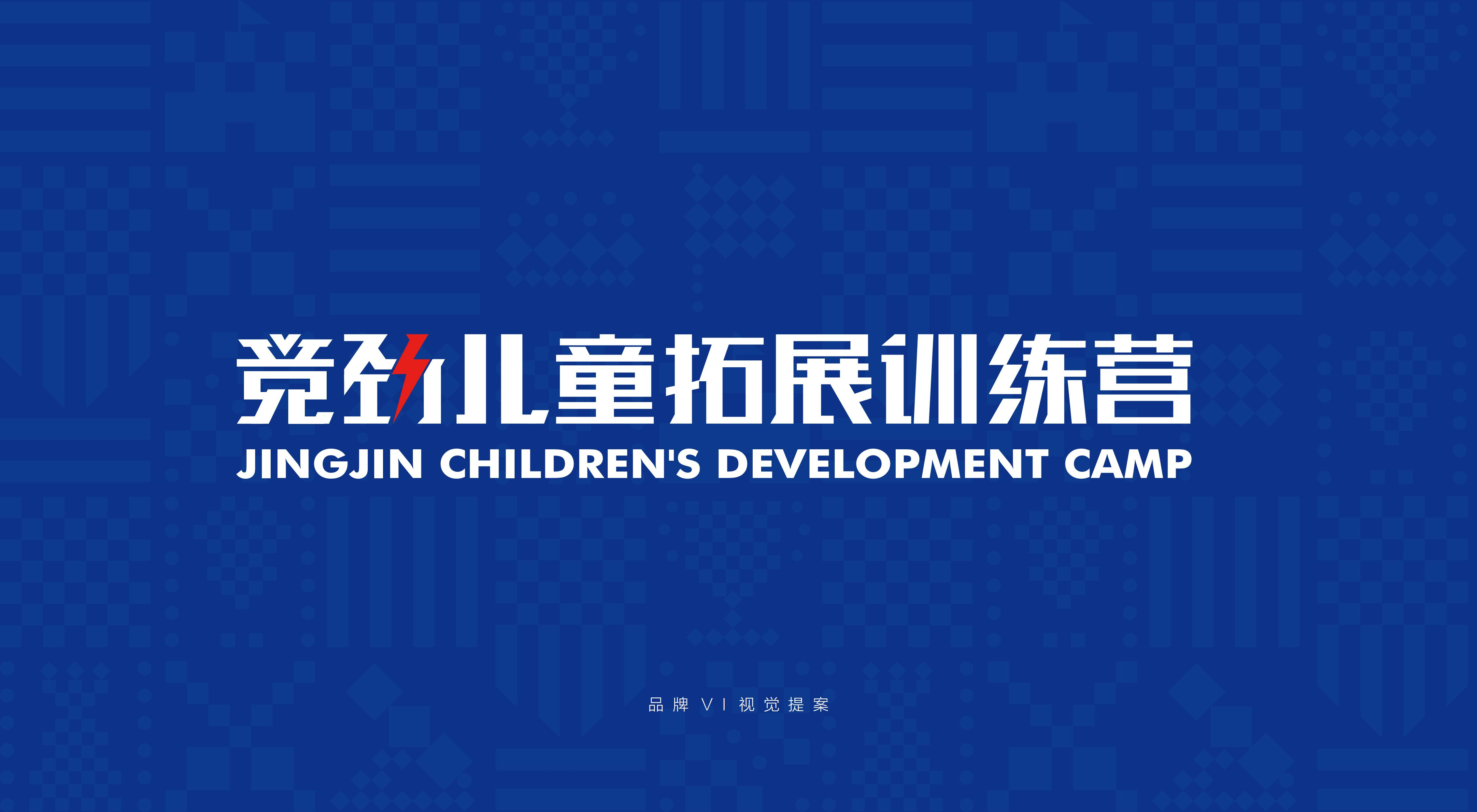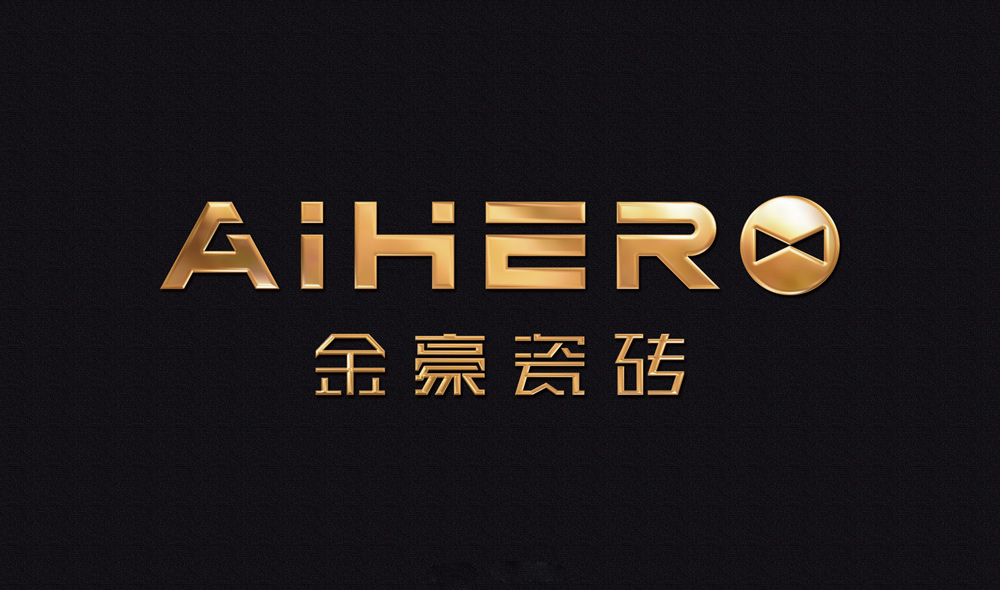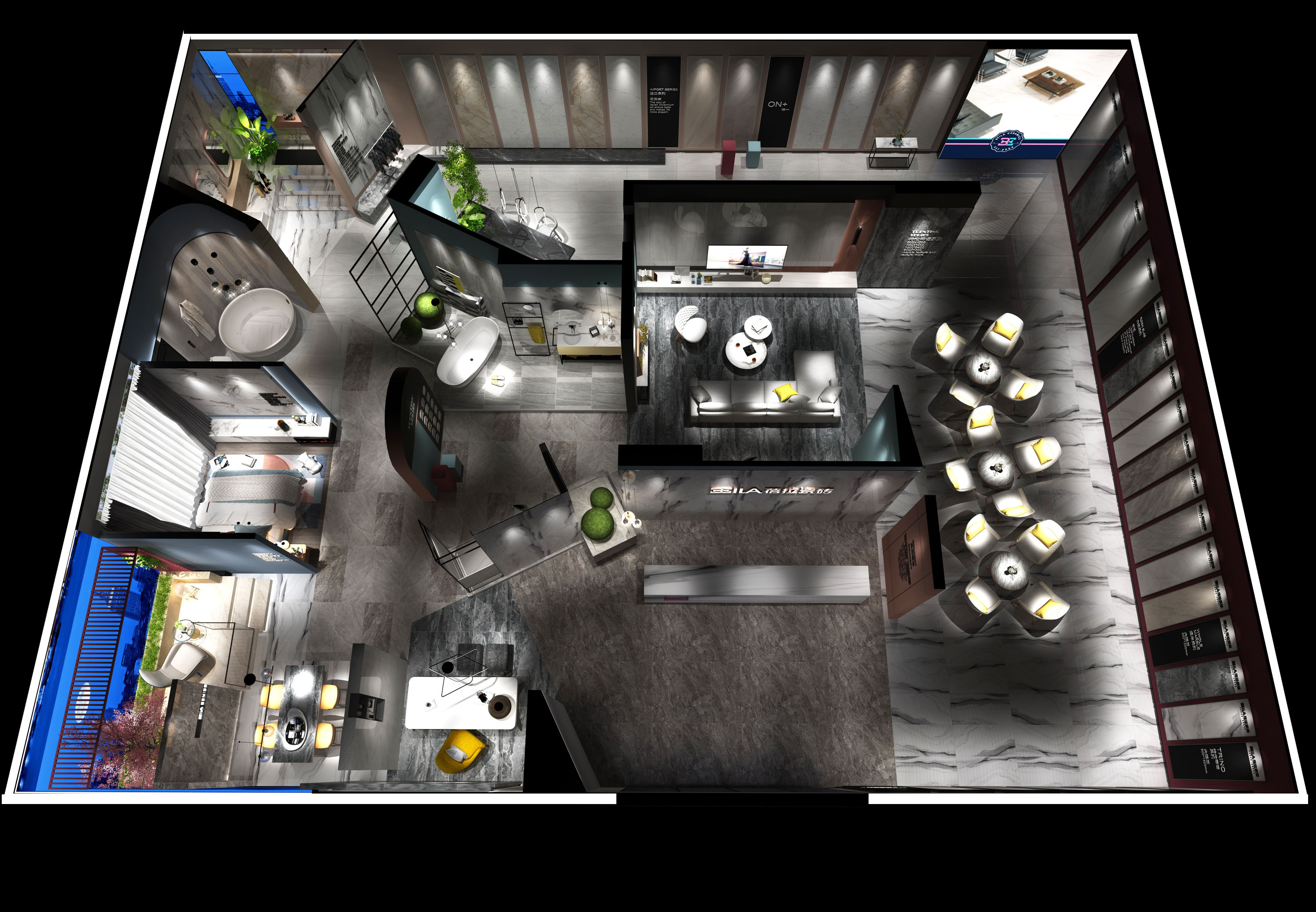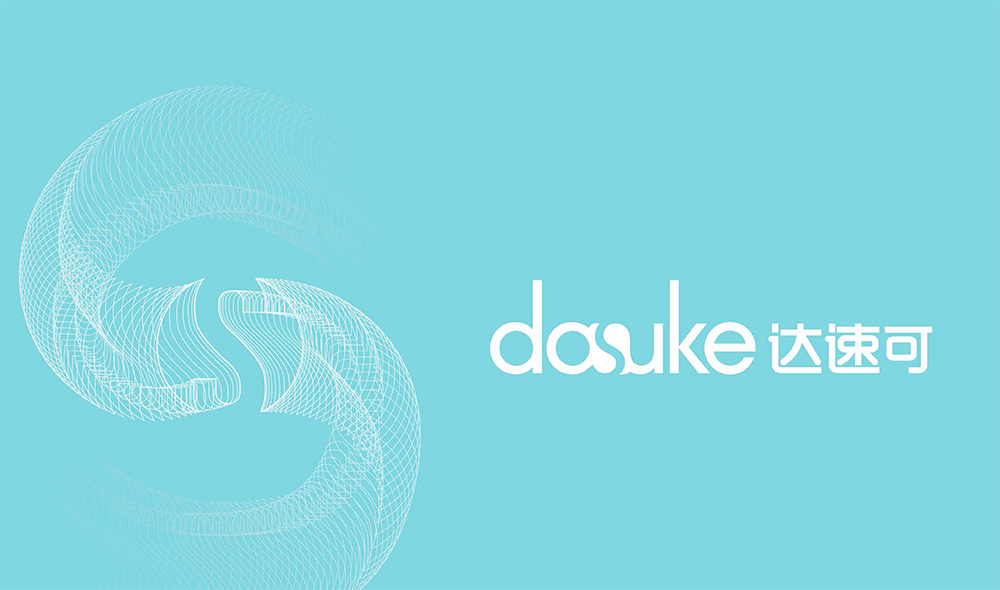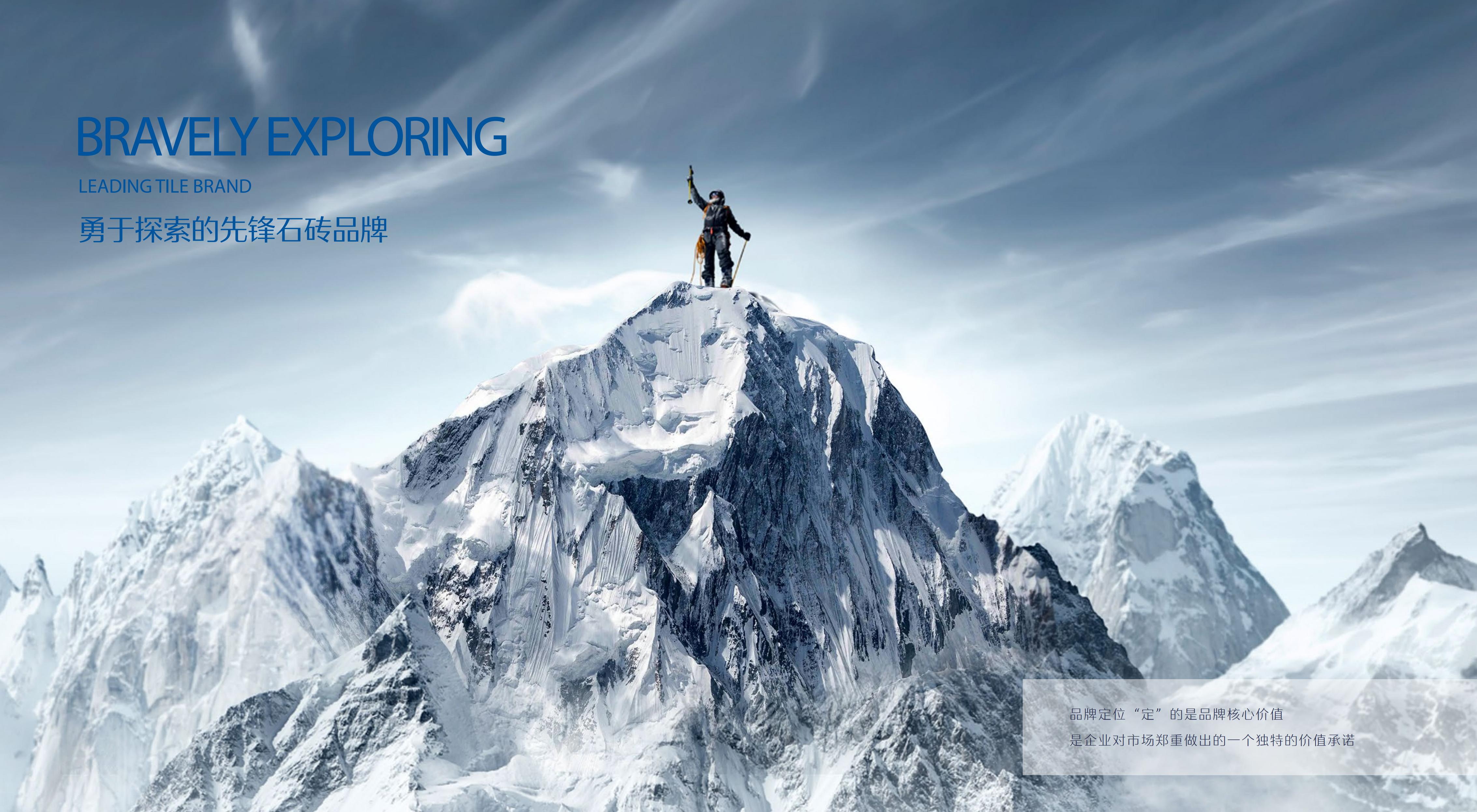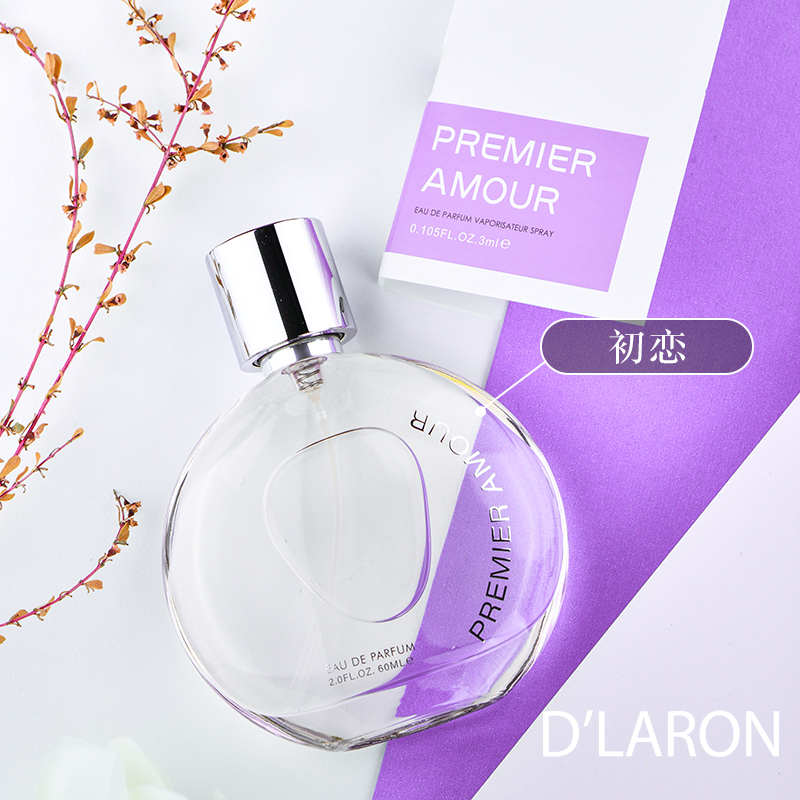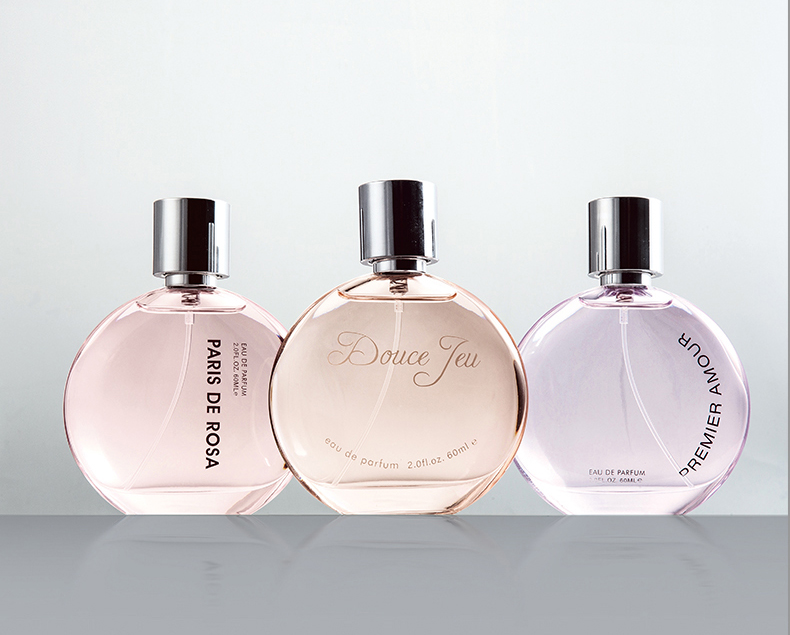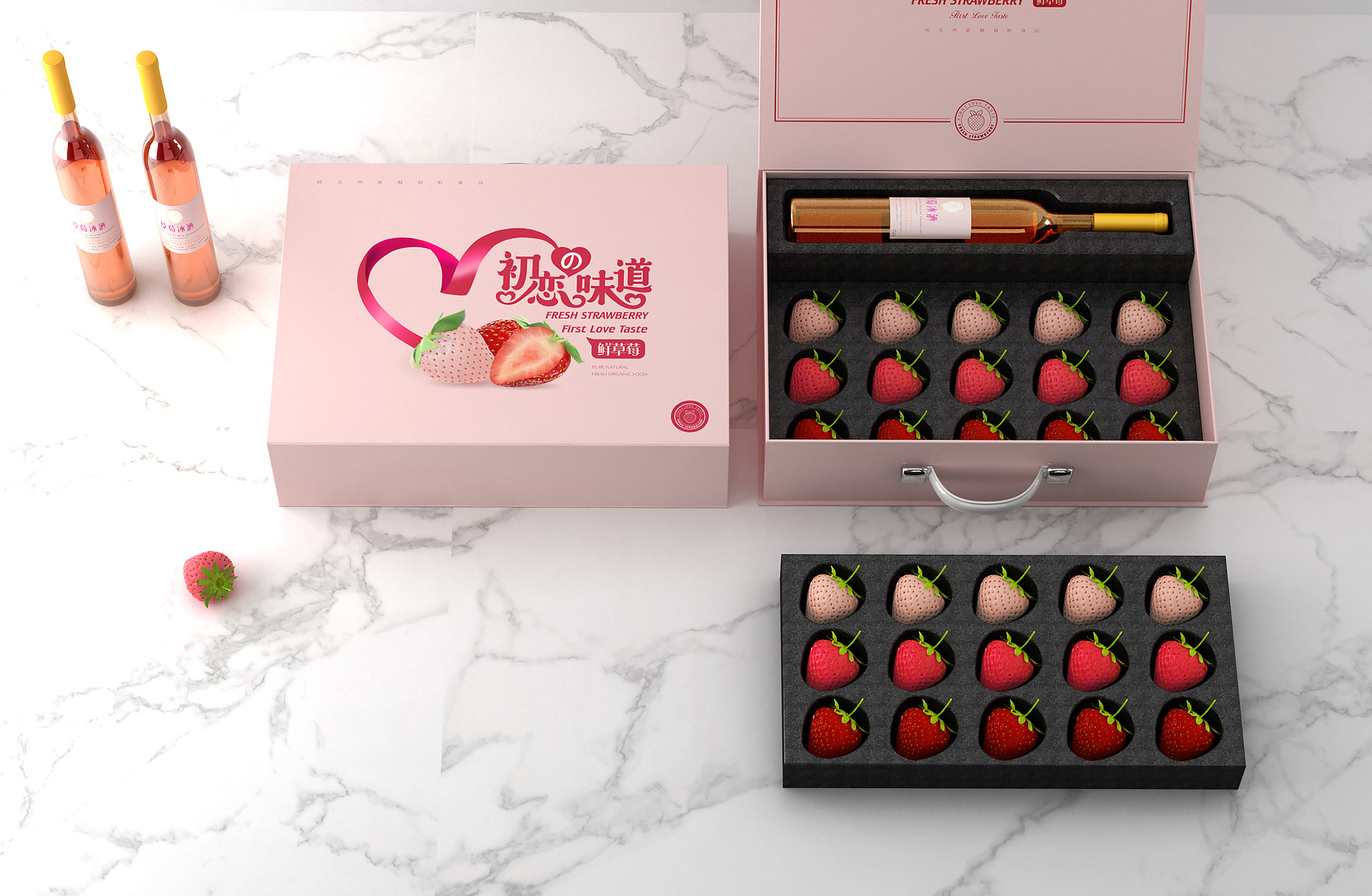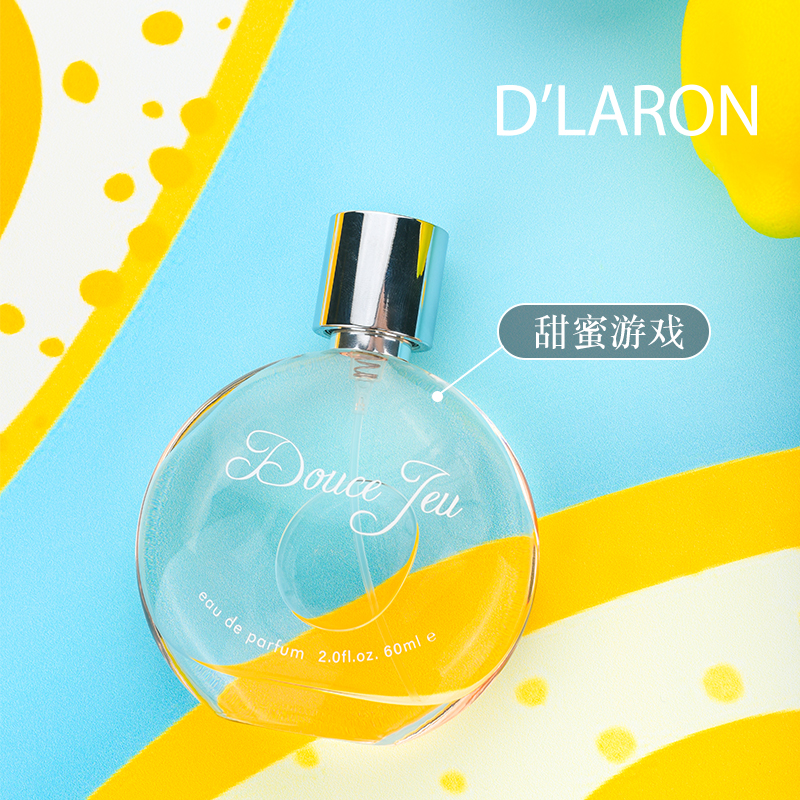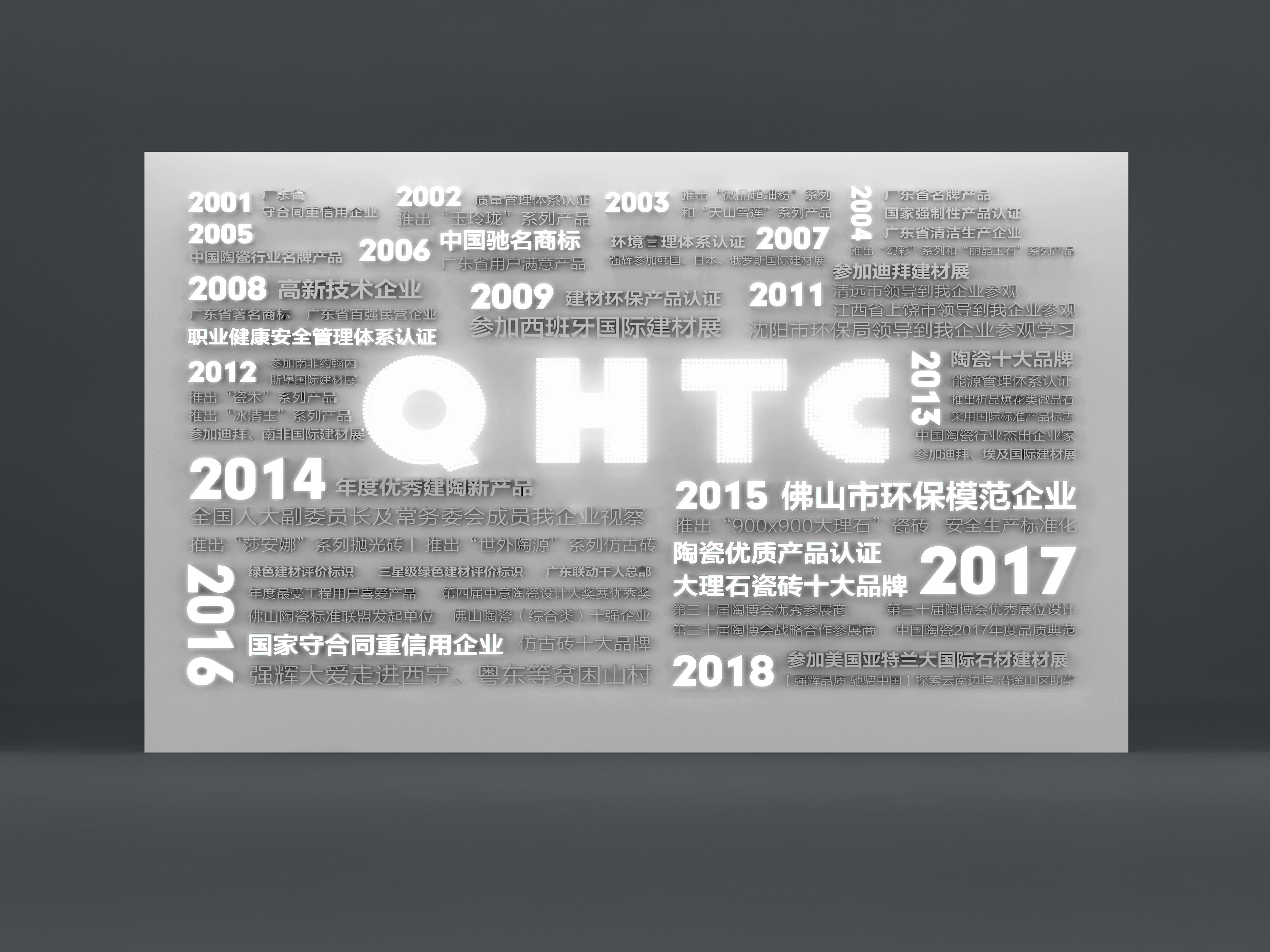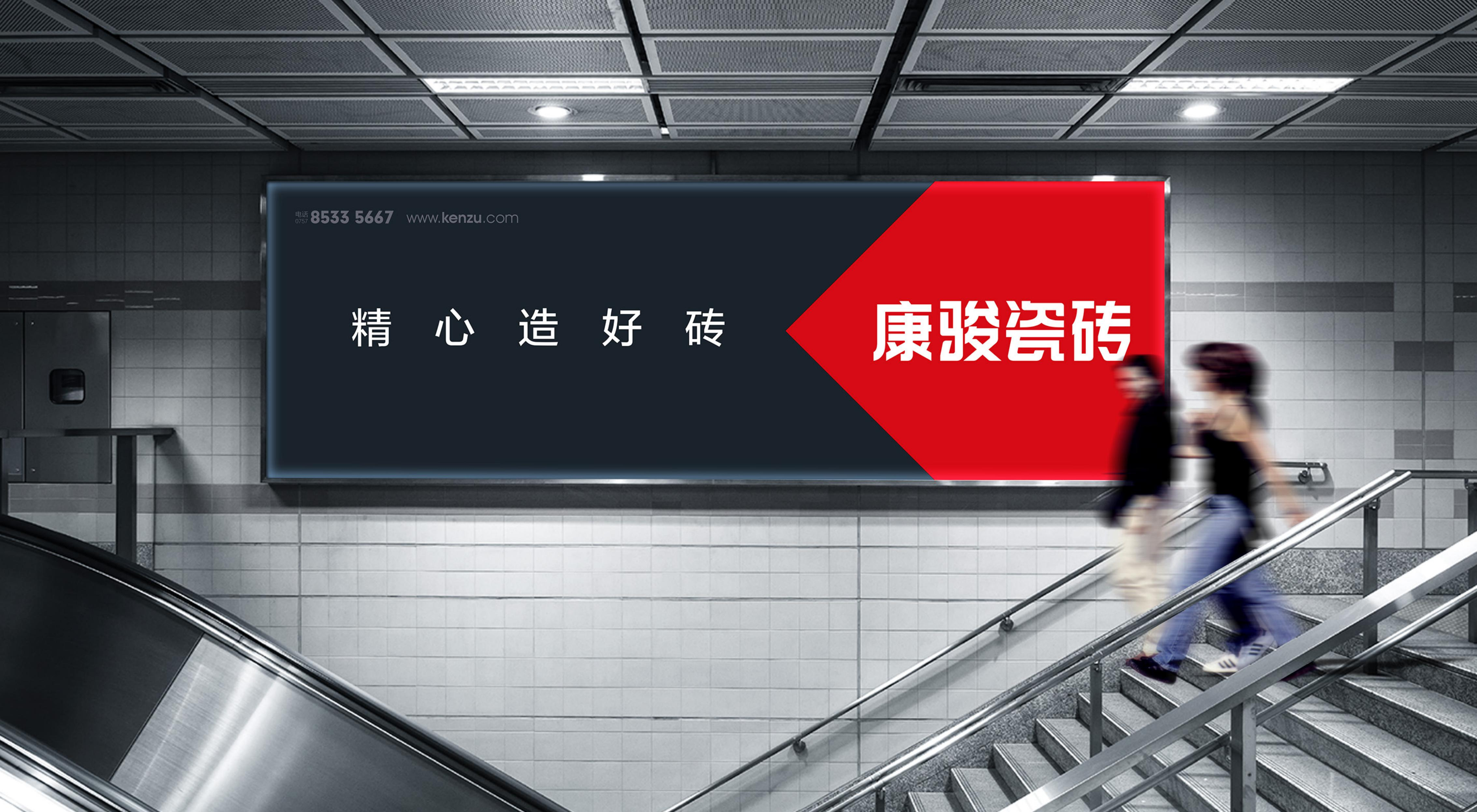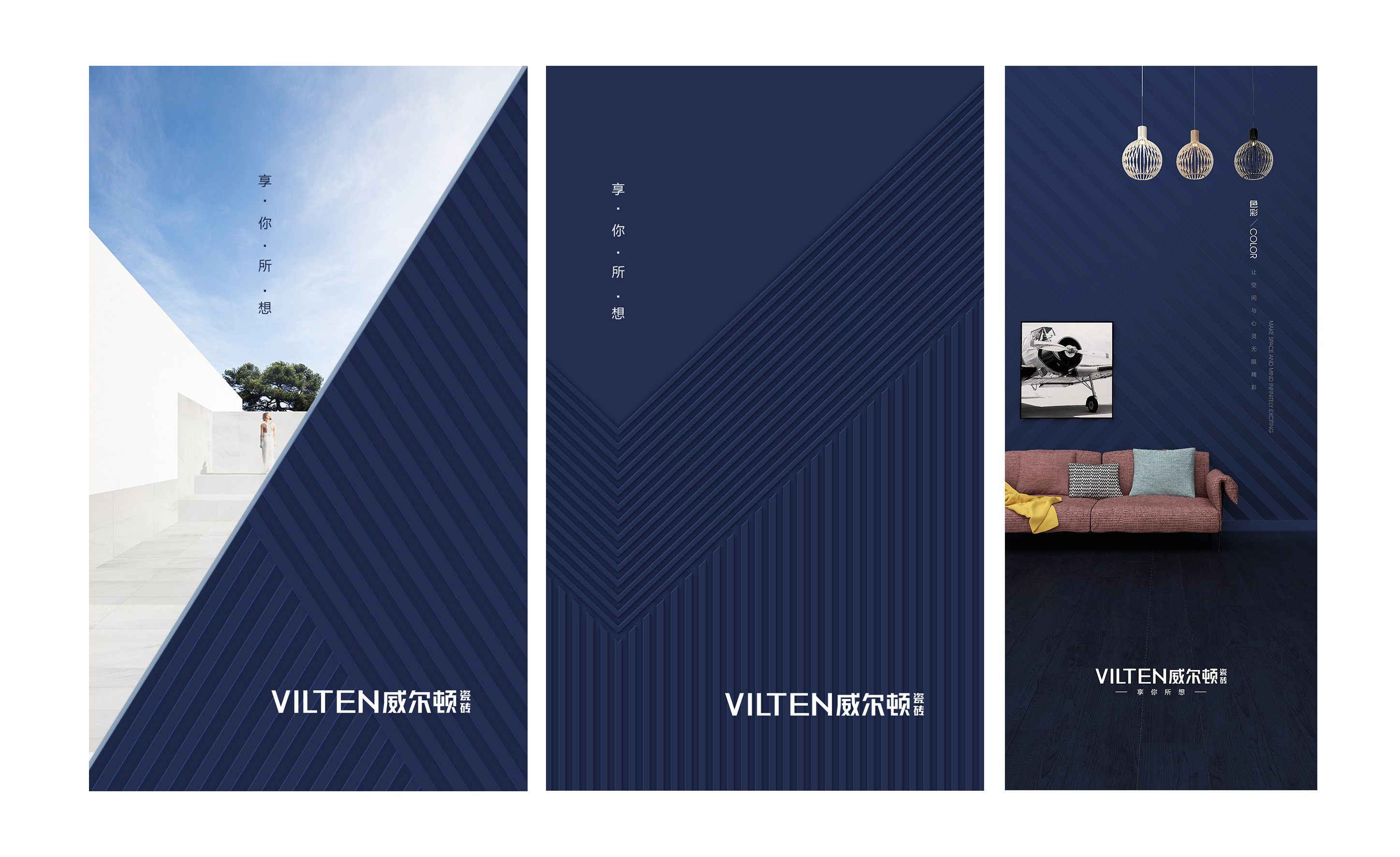178品牌战略创新设计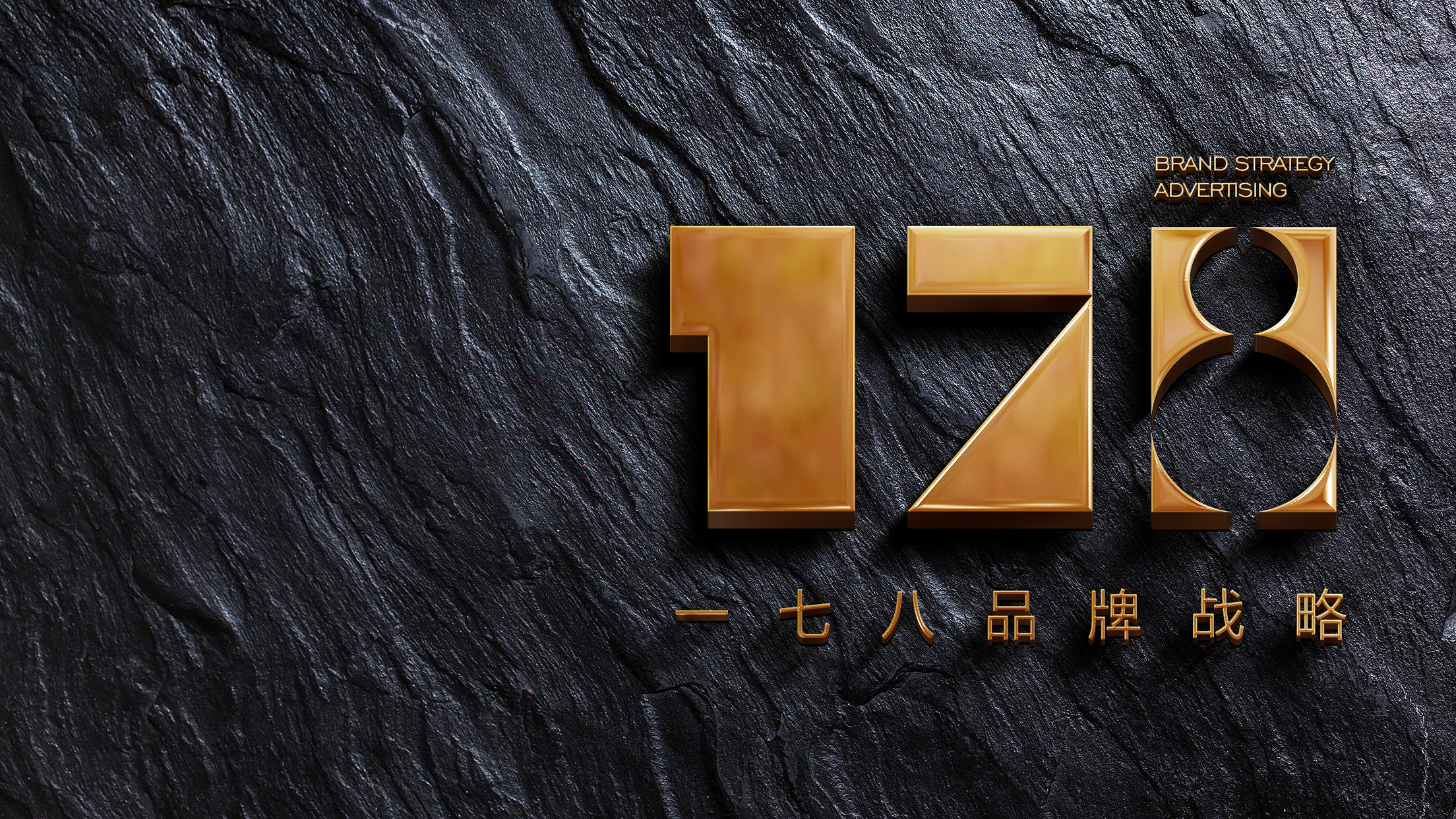•截图，长按识别二维码关注^_^
•截图，长按识别二维码关注^_^
•截图，长按识别二维码关注^_^
•截图，长按识别二维码关注^_^
•截图，长按识别二维码关注^_^
•截图，长按识别二维码关注^_^
•截图，长按识别二维码关注^_^
•截图，长按识别二维码关注^_^
•截图，长按识别二维码关注^_^
•截图，长按识别二维码关注^_^
•截图，长按识别二维码关注^_^
•截图，长按识别二维码关注^_^
•截图，长按识别二维码关注^_^
•截图，长按识别二维码关注^_^
•截图，长按识别二维码关注^_^
•截图，长按识别二维码关注^_^
•截图，长按识别二维码关注^_^
•截图，长按识别二维码关注^_^
•截图，长按识别二维码关注^_^
•截图，长按识别二维码关注^_^
•截图，长按识别二维码关注^_^
•截图，长按识别二维码关注^_^
•截图，长按识别二维码关注^_^
•截图，长按识别二维码关注^_^
•截图，长按识别二维码关注^_^
•截图，长按识别二维码关注^_^
•截图，长按识别二维码关注^_^
•截图，长按识别二维码关注^_^
•截图，长按识别二维码关注^_^
•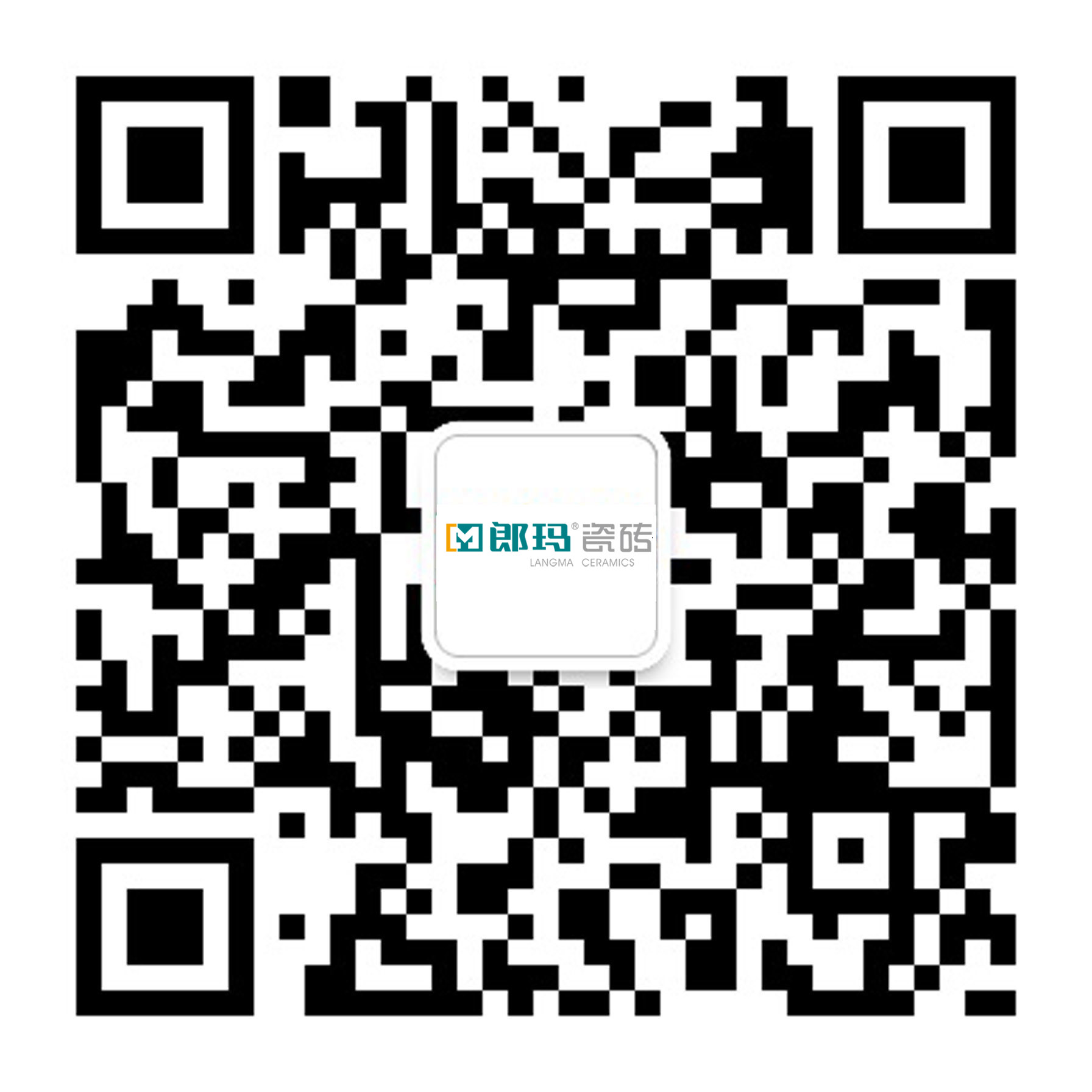截图，长按识别二维码关注^_^
•截图，长按识别二维码关注^_^
•截图，长按识别二维码关注^_^
•截图，长按识别二维码关注^_^
•截图，长按识别二维码关注^_^
•截图，长按识别二维码关注^_^
•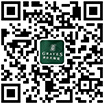截图，长按识别二维码关注^_^
•截图，长按识别二维码关注^_^
•截图，长按识别二维码关注^_^
•截图，长按识别二维码关注^_^
•截图，长按识别二维码关注^_^
•截图，长按识别二维码关注^_^
•截图，长按识别二维码关注^_^
•截图，长按识别二维码关注^_^
•截图，长按识别二维码关注^_^
•截图，长按识别二维码关注^_^